# Abstract Reasoning: 5 Step Strategy For Solving Set A/Set B Questions

Learn how to identify one of the most common Abstract Reasoning patterns

In this article, we’re going to share the 5 step process for solving Ste A/Set B questions.

## Why do I need a strategy for solving Set A/Set B questions?

Students often find Abstract Reasoning to be the most challenging subtest of the UCAT.

However, if you are familiar with the common patterns and rules, it will be easier to identify them under time pressure.## What’s the 5 Step Strategy for solving Set A/Set B Abstract Reasoning Questions?

Remember this 5 step strategy for solving Set A/Set B questions for the Abstract Reasoning subtest:

1. Concentrate on one of the sets first.
2. Start with the simplest square (if there is such a square).
3. Compare the simplest square to the next simplest square(s) to identify the pattern.
4. If you succeed in seeing the pattern, check with the remaining squares to confirm the pattern. If you cannot see the pattern, move on to the other set and repeat steps 1 to 4.
5. The pattern or rules for both sets will be different but of the same type. Once you identify the pattern in one set, you can look for a pattern of the same type in the other set.

One of the most common types of Abstract Reasoning patterns is shown in this example.

## Want a UCAT cheatsheet in your pocket?## Worked example of solving an Abstract Reasoning Set A/Set B Questions in 5 steps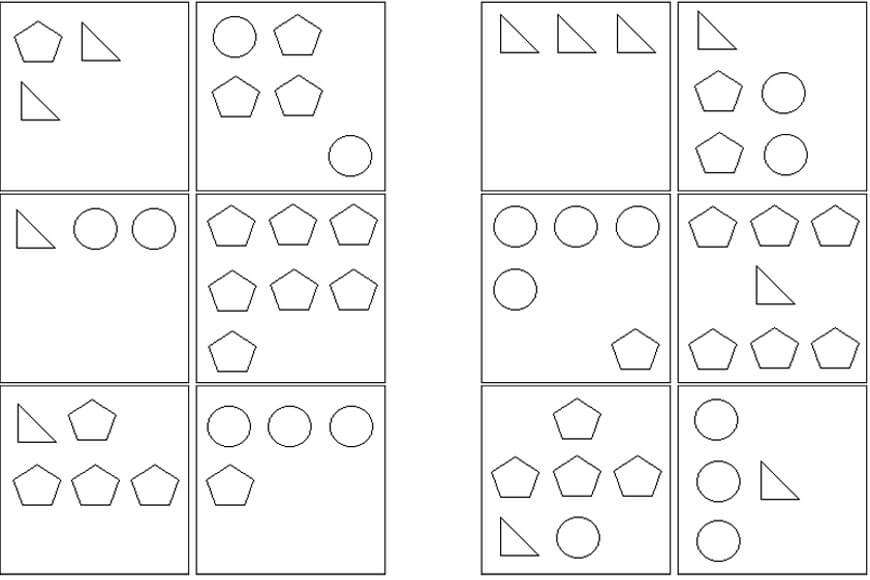### Step 1: Concentrate on one of the sets

Have a go at identifying the pattern and picking whether each of these 5 test shapes fits within set A, set B or neither set.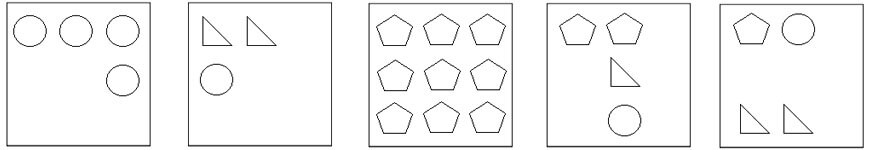Answer: This is an example of an equivalence question where each of the shapes is equivalent to a certain number.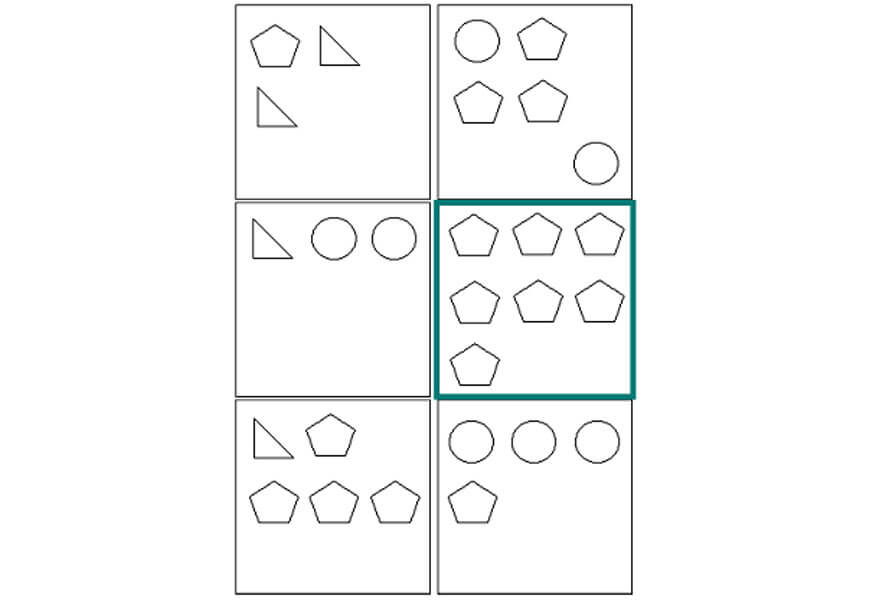### Step 2: Pick the simplest square

First, we start with the simplest square in Set A which is the middle right square.

This is the simplest square because there is only one type of shape in it. There are 7 pentagons, so we can assume that pentagon = 1.

Therefore, the sum of each square must be 7.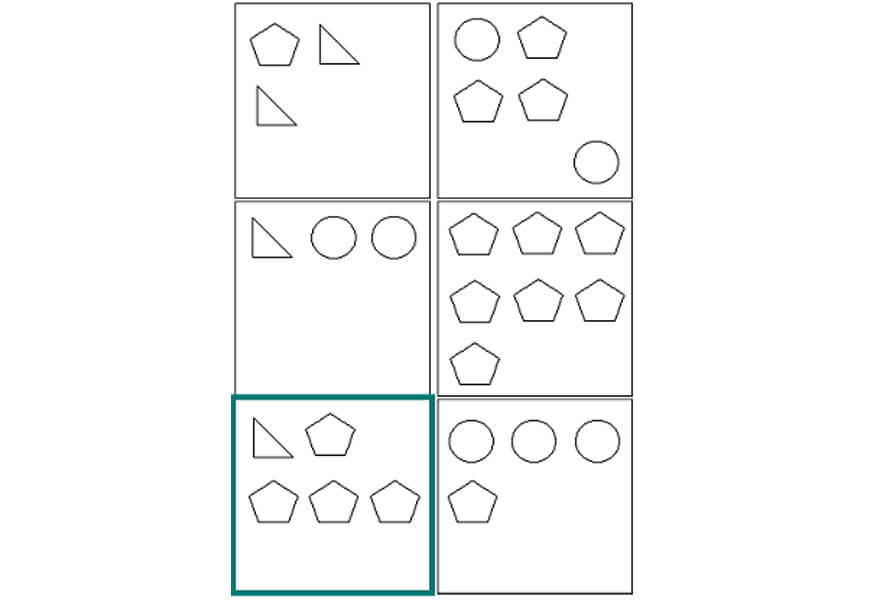### Step 3: Compare the next simplest square

Then, we compare this square to the next simplest square which is the bottom left, because it contains pentagons and one other shape.

If we assume that the sum of each Set A square is 7, there are 4 pentagons so triangle = 3.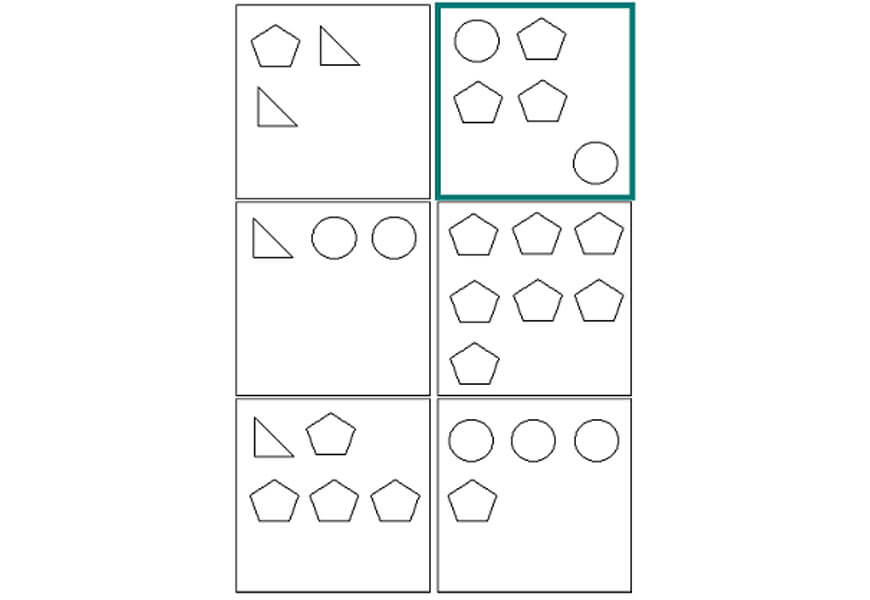### Step 4: Confirm the pattern

Lastly, we can apply the same logic to the top right square. The sum should be 7 and there are 3 pentagons so we can deduce that circle = 2.

### Step 5: Use the pattern from Set A in Set B

If we assign the same values to the pentagon, circle and triangle in set B, we discover that the sum of each square is 9.

To summarise, pentagon = 1, circle = 2 and triangle = 3.
The sum of each square in set A is 7 and the sum of each square in set B is 9.

Test shape 1: There are 4 circles. Sum = 4 x 2 = 8; this fits in neither set.
Test shape 2: There is 1 circle and 2 triangles. Sum = 2 + 2 x 3 = 8; this fits in neither set.
Test shape 3: There are 9 pentagons. Sum = 9 x 1 = 9; this fits in set B.
Test shape 4: There are 2 pentagons, 1 circle and 1 triangle. Sum = 2 x 1 + 2 + 3 = 7; this fits in set A.
Test shape 5: There is 1 pentagon, 1 circle and 2 triangles. Sum = 1 + 2 + 2 x 3 = 9; this fits in set B.